# Create a Waterfall Chart in PowerPoint - Part 2

Explore waterfall chart basics, by learning how to work with positive numbers in PowerPoint.

Author:

Product/Version: PowerPoint

< Continued from Page 1

Continued on Page 3 >

## Inserting a Stacked Column Chart:

Follow these steps to get started:

1. Inserting a Chart: From the Insert tab, select the Chart button, highlighted in red) within Figure 1, below.
2.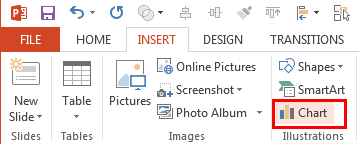Figure 1: Select the Chart button
3. Selecting the Stacked Column chart: From within the Insert Chart dialog box, select the Stacked Column chart (this is what we will manipulate to create the Waterfall chart), which inserts the chart onto your slide and opens up an Excel spreadsheet.
4.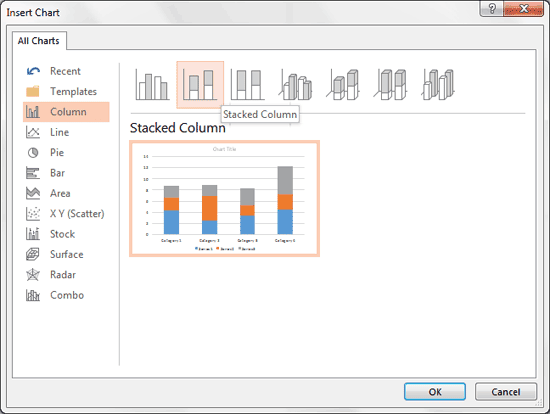Figure 2: Select Stacked Column Chart
5. Note: Be sure to select the Stacked Column chart, and not the similarly-named 100% Stacked Column chart.

## Setting up the Numbers:

The most important step to building a Waterfall chart is setting up the numbers in Excel correctly. Throughout this tutorial, I will use the below data points to create the Waterfall Chart (see Figure 3).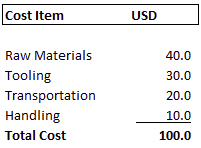Figure 3: Data points to create the Waterfall Chart

1. Prepping the Spreadsheet: With the above data inserted into the Excel spreadsheet starting at cell A8, the first step is to calculate the three data points we need for the Waterfall chart; what I will refer to as the Base, the Value and the Total (see Figure 4 below). I recommend always calculating them to the right of your original data points.
2.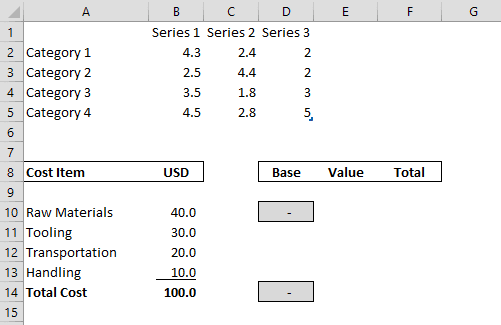Figure 4: Calculate the data points
3. Note: I've formatted the first and last numbers in the Base column (D10 and D14) with a grey fill, and hardcoded the number 0 into each cell. Whenever building Waterfall Charts, the first, last and any in-between sub-totals, always need to start with a base value of zero.
4. Calculating the Value: Let's continue with the first row of information for our Waterfall chart. We want to make the Value equal to our original number, so in cell E10, type in the formula "=B10" as shown in Figure 5 below.
5.Figure 5: In cell E10, type in the formula "=B10"
6. Calculating the Total: For the Total, we simply want to add up the Base and the Value. So in cell F10, type the formula "=E10 + D10" as shown in Figure 6 below.
7.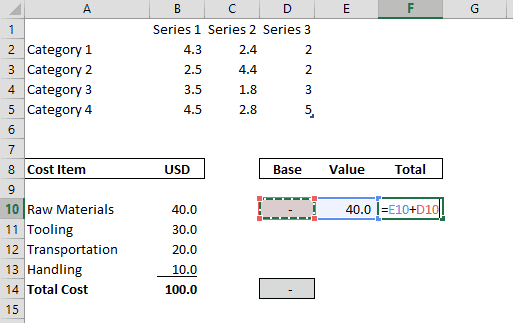Figure 6: Adding the Base and the Value
8. Filling in the Values and Totals: With the Value and Total calculated for the first row, we can then fill in the rest of the Values and Totals. So selecting the first two cells, E10 and F10, copy the formula down to row 14. You can do this by selecting the small square in the lower right-hand corner and dragging it down to F14 (see Figure 7).
9.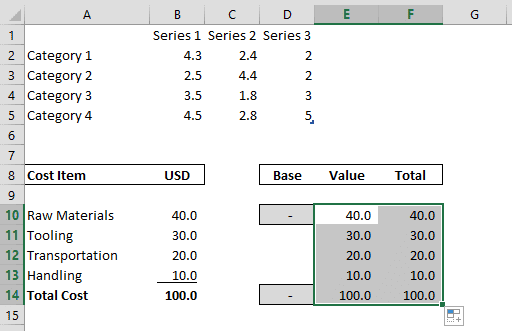Figure 7: Copy the formula down to row 14
10. Calculating the 'Base': For the remaining Base numbers, we want to make them each equal to the previous data point's Total. So select cells D11 through D13, and type in the formula "=F10".
11.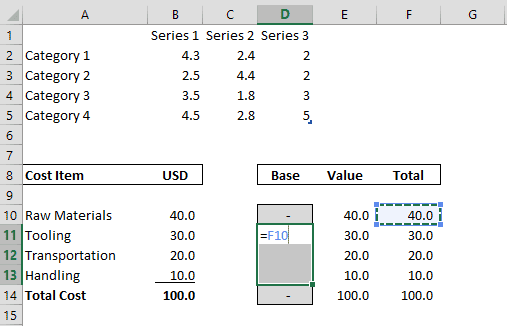Figure 8: Select cells D11 through D13
12. And then holding the Ctrl key down, hit Enter, to input the formula into all of the selected cells, as shown in Figure 9, below.
13.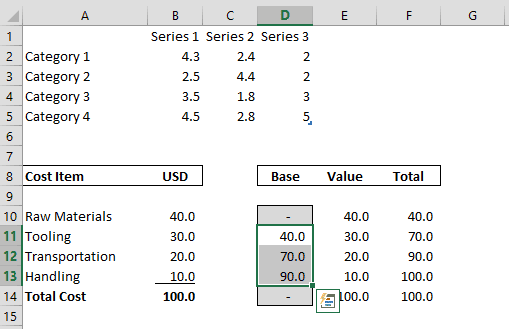Figure 9: Hold Ctrl and hit Enter

Bringing the Information Into Our Chart, continued on Page 3 >

### Popular PostsApplying Slide Masters to Individual Slides in PowerPoint 2010
Learn how to apply Slide Masters to individual slides in PowerPoint 2010.Copy and Remove Highlighting for Text in PowerPoint 2013
Learn how to copy and remove highlighting for text in PowerPoint 2013.Learn how to add Headers and Footers to slides in PowerPoint 2016 for Windows.Text Box Autofit Options in PowerPoint 2016 for Windows
Learn about Text Box autofit options in PowerPoint 2016.

## Pictures in Presentations

Is a picture is worth a thousand words? You probably have heard this adage so often that we decided not to repeat this phrase throughout this book! Now here’s some more info: the human brain uses a larger part of its area to store visual information rather than textual content. And that’s possibly because a picture describes so much more than text.

Go and get a copy of our Pictures in Presentations ebook.

This is the original page. An AMP (Accelerated Mobile Page) version of this page is also available for those on mobile platforms, at Create a Waterfall Chart in PowerPoint - Part 2.

Microsoft and the Office logo are trademarks or registered trademarks of Microsoft Corporation in the United States and/or other countries.

Plagiarism will be detected by Copyscape